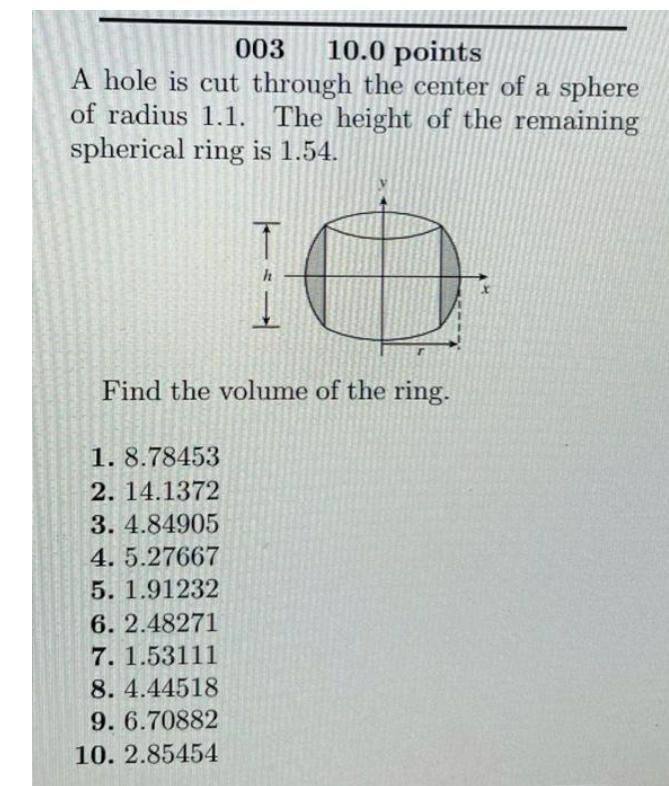Question:

# A hole is cut through the center of a sphere of radius 1.1. The height of the remaining spherical ring is 1.54. Find the volume of the ring. 1). 8.78453 2). 14.1372 3). 4.84905 4). 5.27667 5). 1.9123A hole is cut through the center of a sphere of radius 1.1. The height of the remaining spherical ring is 1.54. Find the volume of the ring. 1). 8.78453 2). 14.1372 3). 4.84905 4). 5.27667 5). 1.91232 6). 2.48271 7). 1.53111 8). 4.44518 9). 6.70882 10).2.85454